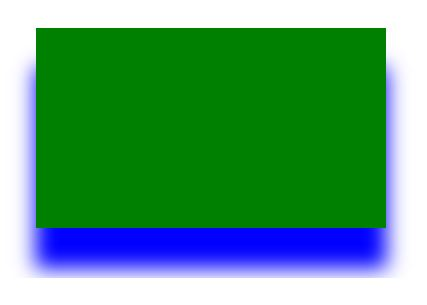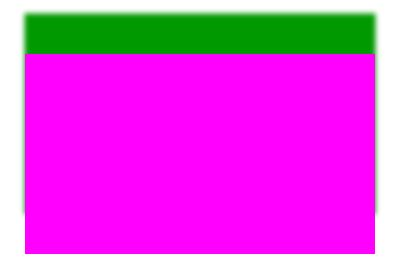# HTML | canvas shadowOffsetY Property

The canvas shadowOffsetY property is used to set or return the vertical distance of the shadow from the shape.
This property holds a positive or negative value where a positive value indicates the shadow to the bottom and a negative value indicates the shadow to the top.

Syntax:

`context.shadowOffsetY = number`

Property Values:

• number: It is used to set a positive or negative number which represents the vertical distance of shadow.

Example-1:

 ` ` `<``html``> ` ` `  `<``head``> ` `    ``<``title``> ` `        ``HTML canvas shadowOffsetY Property ` `    `` ` ` ` ` `  `<``body``> ` `    ``<``canvas` `id``=``"GFG"` `            ``width``=``"500"`  `            ``height``=``"300"``> ` `  `` ` ` `  `    ``<``script``> ` `        ``var x = ` `            ``document.getElementById("GFG"); ` `        ``var contex = ` `            ``x.getContext("2d"); ` `        ``contex.shadowBlur = 20; ` `       `  `        ``// vertical distance of shadow ` `        ``contex.shadowOffsetY = 40; ` `        ``contex.shadowColor = "blue"; ` `        ``contex.fillStyle = "green"; ` `        ``contex.fillRect(50, 50, 350, 200); ` `        ``contex.stroke(); ` `    `` ` ` `  ` ` ` `  ` `

Output:Example-2:

 ` ` `<``html``> ` ` `  `<``head``> ` `    ``<``title``> ` `        ``HTML canvas shadowOffsetY Property ` `    `` ` ` ` ` `  `<``body``> ` `    ``<``canvas` `id``=``"GFG"`  `            ``width``=``"500"` `            ``height``=``"300"``> ` `  `` ` ` `  `    ``<``script``> ` `        ``var x = ` `            ``document.getElementById("GFG"); ` `        ``var contex = ` `            ``x.getContext("2d"); ` `        ``contex.shadowBlur = 5; ` ` `  `        ``// vertical distance of shadow. ` `        ``contex.shadowOffsetY = -40; ` `        ``contex.shadowColor = "rgb(0, 153, 0)"; ` `        ``contex.fillStyle = "rgb(255, 0, 255)"; ` `        ``contex.fillRect(50, 50, 350, 200); ` `        ``contex.stroke(); ` `    `` ` ` `  ` ` ` `  ` `

Output:Supported Browsers:

• Internet Explorer 9.0
• Firefox
• Safari
• OperaMy Personal Notes arrow_drop_upCheck out this Author's contributed articles.

If you like GeeksforGeeks and would like to contribute, you can also write an article using contribute.geeksforgeeks.org or mail your article to contribute@geeksforgeeks.org. See your article appearing on the GeeksforGeeks main page and help other Geeks.

Please Improve this article if you find anything incorrect by clicking on the "Improve Article" button below.

Article Tags :
Practice Tags :

Be the First to upvote.

Please write to us at contribute@geeksforgeeks.org to report any issue with the above content.